Limit TheoremsCustom SearchLIMIT THEOREMS To obtain results in calculus, we will frequently operate with limits. The proofs of theorems shown in this section will be omitted in the interest of brevity. The theorems will be stated and examples will be given. Assume that we have three simple functions of x. Further, let these functions [ f (x), g(x), and h (x)] have separate limits such thatTheorem 1. The limit of the sum of two functions is equal to the sum of the limits:This theorem may be extended to include any number of functions, such asEXAMPLE: Find the limit ofSOLUTION: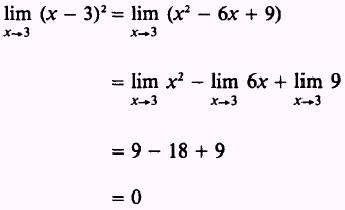Theorem 2. The limit of a constant, c, times a function, f(x), is equal to the constant, c, times the limit of the function: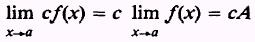EXAMPLE: Find the limit of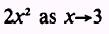SOLUTIONŁ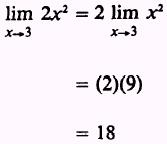Theorem 3. The limit of the product of two functions is equal to the product of their limits:EXAMPLE: Find the limit of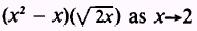SOLUTION.Theorem 4. The limit of the quotient of two functions is equal to the quotient of their limits, provided the limit of the divisor is not equal to zero: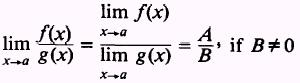EXAMPLE: Find the limit of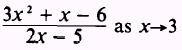SOLUTIONŁ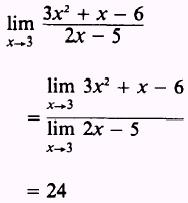PRACTICE PROBLEMS Find the limits of the following, using the theorem indicated: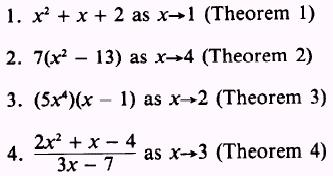ANSWERS:Integrated Publishing, Inc. - A (SDVOSB) Service Disabled Veteran Owned Small Business